# Jacob Bernoulli facts for kids

Kids Encyclopedia Facts
Quick facts for kids
Jacob Bernoulli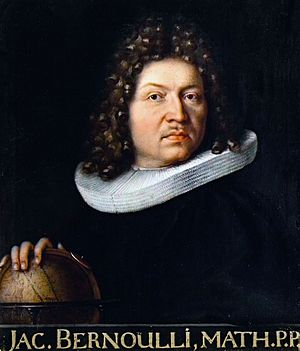Born 6 January 1655
Basel, Switzerland
Died 16 August 1705 (aged 50)
Basel, Switzerland
Education University of Basel
(D.Th., 1676; Dr. phil. hab., 1684)
Known for Bernoulli differential equation
Bernoulli numbers
Bernoulli's formula
Bernoulli polynomials
Bernoulli map
Bernoulli trial
Bernoulli process
Bernoulli scheme
Bernoulli operator
Hidden Bernoulli model
Bernoulli sampling
Bernoulli distribution
Bernoulli random variable
Bernoulli's Golden Theorem
Bernoulli's inequality
Lemniscate of Bernoulli
Scientific career
Fields Mathematics, mechanics
Institutions University of Basel
Theses
• Primi et Secundi Adami Collatio (1676)
• Solutionem tergemini problematis arithmetici, geometrici et astronomici (Solutions to a triple problem in arithmetics, geometry and astronomy) (1684)
Doctoral students Jacob Hermann
Nicolaus I Bernoulli
Other notable students Johann Bernoulli
Influences Nicolas Malebranche
Notes
Brother of Johann Bernoulli

Jacob Bernoulli (also known as James or Jacques; 6 January 1655 [O.S. 27 December 1654] – 16 August 1705) was one of the many prominent mathematicians in the Bernoulli family. He was an early proponent of Leibnizian calculus and sided with Gottfried Wilhelm Leibniz during the Leibniz–Newton calculus controversy. He is known for his numerous contributions to calculus, and along with his brother Johann, was one of the founders of the calculus of variations. He also discovered the fundamental mathematical constant e. However, his most important contribution was in the field of probability, where he derived the first version of the law of large numbers in his work Ars Conjectandi.

## Biography

Jacob Bernoulli was born in Basel, Switzerland. Following his father's wish, he studied theology and entered the ministry. But contrary to the desires of his parents, he also studied mathematics and astronomy. He traveled throughout Europe from 1676 to 1682, learning about the latest discoveries in mathematics and the sciences under leading figures of the time. This included the work of Johannes Hudde, Robert Boyle, and Robert Hooke. During this time he also produced an incorrect theory of comets.

Bernoulli returned to Switzerland, and began teaching mechanics at the University of Basel from 1683. His doctoral dissertation Solutionem tergemini problematis was submitted in 1684. It appeared in print in 1687.

In 1684 Bernoulli married Judith Stupanus; they had two children. During this decade, he also began a fertile research career. His travels allowed him to establish correspondence with many leading mathematicians and scientists of his era, which he maintained throughout his life. During this time, he studied the new discoveries in mathematics, including Christiaan Huygens's De ratiociniis in aleae ludo, Descartes' La Géométrie and Frans van Schooten's supplements of it. He also studied Isaac Barrow and John Wallis, leading to his interest in infinitesimal geometry. Apart from these, it was between 1684 and 1689 that many of the results that were to make up Ars Conjectandi were discovered.

He was appointed professor of mathematics at the University of Basel in 1687, remaining in this position for the rest of his life. By that time, he had begun tutoring his brother Johann Bernoulli on mathematical topics. The two brothers began to study the calculus as presented by Leibniz in his 1684 paper on the differential calculus in "Nova Methodus pro Maximis et Minimis" published in Acta Eruditorum. They also studied the publications of von Tschirnhaus. It must be understood that Leibniz's publications on the calculus were very obscure to mathematicians of that time and the Bernoullis were among the first to try to understand and apply Leibniz's theories.

Jacob collaborated with his brother on various applications of calculus. However the atmosphere of collaboration between the two brothers turned into rivalry as Johann's own mathematical genius began to mature, with both of them attacking each other in print, and posing difficult mathematical challenges to test each other's skills. By 1697, the relationship had completely broken down.

The lunar crater Bernoulli is also named after him jointly with his brother Johann.

## Important works

Jacob Bernoulli's first important contributions were a pamphlet on the parallels of logic and algebra published in 1685, work on probability in 1685 and geometry in 1687. His geometry result gave a construction to divide any triangle into four equal parts with two perpendicular lines.

By 1689 he had published important work on infinite series and published his law of large numbers in probability theory. Jacob Bernoulli published five treatises on infinite series between 1682 and 1704. The first two of these contained many results, such as the fundamental result that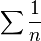$\sum{\frac{1}{n}}$ diverges, which Bernoulli believed were new but they had actually been proved by Pietro Mengoli 40 years earlier and was proved by Nicole Oresme in the 14th century already. Bernoulli could not find a closed form for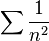$\sum{\frac{1}{n^2}}$, but he did show that it converged to a finite limit less than 2. Euler was the first to find the limit of this series in 1737. Bernoulli also studied the exponential series which came out of examining compound interest.

In May 1690 in a paper published in Acta Eruditorum, Jacob Bernoulli showed that the problem of determining the isochrone is equivalent to solving a first-order nonlinear differential equation. The isochrone, or curve of constant descent, is the curve along which a particle will descend under gravity from any point to the bottom in exactly the same time, no matter what the starting point. It had been studied by Huygens in 1687 and Leibniz in 1689. After finding the differential equation, Bernoulli then solved it by what we now call separation of variables. Jacob Bernoulli's paper of 1690 is important for the history of calculus, since the term integral appears for the first time with its integration meaning. In 1696 Bernoulli solved the equation, now called the Bernoulli differential equation,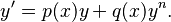$y' = p(x)y + q(x)y^n.$

Jacob Bernoulli also discovered a general method to determine evolutes of a curve as the envelope of its circles of curvature. He also investigated caustic curves and in particular he studied these associated curves of the parabola, the logarithmic spiral and epicycloids around 1692. The lemniscate of Bernoulli was first conceived by Jacob Bernoulli in 1694. In 1695 he investigated the drawbridge problem which seeks the curve required so that a weight sliding along the cable always keeps the drawbridge balanced.

Bernoulli's most original work was Ars Conjectandi, published in Basel in 1713, eight years after his death. The work was incomplete at the time of his death but it is still a work of the greatest significance in the theory of probability. The book also covers other related subjects, including a review of combinatorics, in particular the work of van Schooten, Leibniz, and Prestet, as well as the use of Bernoulli numbers in a discussion of the exponential series. Inspired by Huygens' work, Bernoulli also gives many examples on how much one would expect to win playing various games of chance. The term Bernoulli trial resulted from this work.

In the last part of the book, Bernoulli sketches many areas of mathematical probability, including probability as a measurable degree of certainty; necessity and chance; moral versus mathematical expectation; a priori an a posteriori probability; expectation of winning when players are divided according to dexterity; regard of all available arguments, their valuation, and their calculable evaluation; and the law of large numbers.

Bernoulli was one of the most significant promoters of the formal methods of higher analysis. Astuteness and elegance are seldom found in his method of presentation and expression, but there is a maximum of integrity.

## Discovery of the mathematical constant e

In 1683 Bernoulli discovered the constant e by studying a question about compound interest which required him to find the value of the following expression (which is in fact e):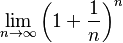$\lim_{n\to\infty} \left( 1 + \frac{1}{n} \right)^n$

One example is an account that starts with $1.00 and pays 100 percent interest per year. If the interest is credited once, at the end of the year, the value is$2.00; but if the interest is computed and added twice in the year, the $1 is multiplied by 1.5 twice, yielding$1.00×1.5² = $2.25. Compounding quarterly yields$1.00×1.254 = $2.4414..., and compounding monthly yields$1.00×(1.0833...)12 = $2.613035.... Bernoulli noticed that this sequence approaches a limit (the force of interest) for more and smaller compounding intervals. Compounding weekly yields$2.692597..., while compounding daily yields $2.714567..., just two cents more. Using n as the number of compounding intervals, with interest of 100% / n in each interval, the limit for large n is the number that Euler later named e; with continuous compounding, the account value will reach$2.7182818.... More generally, an account that starts at \$1, and yields (1+R) dollars at compound interest, will yield eR dollars with continuous compounding.

## Tombstone

Bernoulli wanted a logarithmic spiral and the motto Eadem mutata resurgo ('Although changed, I rise again the same') engraved on his tombstone. He wrote that the self-similar spiral "may be used as a symbol, either of fortitude and constancy in adversity, or of the human body, which after all its changes, even after death, will be restored to its exact and perfect self." Bernoulli died in 1705, but an Archimedean spiral was engraved rather than a logarithmic one.

Translation of Latin inscription:

Jacob Bernoulli, the incomparable mathematician.
Professor at the University of Basel For more than 18 years;
member of the Royal Academies of Paris and Berlin; famous for his writings.
Of a chronic illness, of sound mind to the end;
succumbed in the year of grace 1705, the 16th of August, at the age of 50 years and 7 months, awaiting the resurrection.
Judith Stupanus,
his wife for 20 years,
and his two children have erected a monument to the husband and father they miss so much.

## Works

• (in la) Conamen novi systematis cometarum. Amstelaedami: apud Henr. Wetstenium. 1682. https://gutenberg.beic.it/webclient/DeliveryManager?pid=17384&custom_att_2=simple_viewer&search_terms=DTL4&pds_handle=. (title roughly translates as "A new hypothesis for the system of comets".)
• (in la) De gravitate aetheris. Amstelaedami: apud Henricum Wetstenium. 1683. https://gutenberg.beic.it/webclient/DeliveryManager?pid=1216514&custom_att_2=simple_viewer&search_terms=DTL5&pds_handle=.
• Ars conjectandi, opus posthumum, Basileae, impensis Thurnisiorum Fratrum, 1713.
• (in la) Opera. 1. Genève: héritiers Cramer & frères Philibert. 1744. https://gutenberg.beic.it/webclient/DeliveryManager?pid=12199963.
• (in la) Opera. 2. Genève: héritiers Cramer & frères Philibert. 1744. https://gutenberg.beic.it/webclient/DeliveryManager?pid=12202240.In Spanish: Jakob Bernoulli para niños